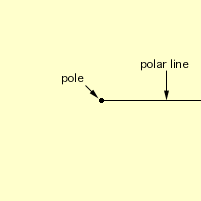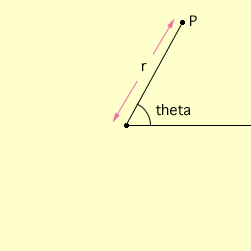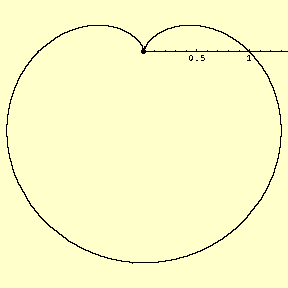Name: Courtney Dohoney Who is asking: Student Level: Secondary Question: Hello, I was assigned a valentines project. Our job is to make a valentines card that has a math theme. On my valentines card I would like to include the set of equations that when graphed look like a heart. If you can figure out a set of equations and get it back to me I would be very happy. Thank you for your time. Sincerely, Courtney Dohoney Hi Courtney, There is r nice way to plot a heart but it involves a way of putting a coordinate system which is different from the usual Cartesian coordinate system. It is call the polar coordinate system. This system starts with a point called the pole and a ray starting at the pole and extending to infinite. Usually we draw this ray, called the polar line, horizontal and extending to the right of the pole.The pole is given coordinates (0,0). Each other point P in the plane is given coordinates (r,theta) as follows. Draw a line segment from P to the pole. r is the length of this line segment Theta is the angle, measured counterclockwise, from the polar line to the line segment joining P to the poleUsing polar coordinates some graphs are very easy to describe. For example the expression r = 3 describes all points in the plane which are 3 units from the pole. Hence the graph of r = 3 is the circle with center at the pole and radius 3. It takes a little practice to graph in polar coordinates but Tthe graph of the polar coordinate equation r = -(1 + sin(theta)) is shown below.So with a little red paint it looks likeThis curve is called a cardiod from th Greek word kardioeides meaning heart-shaped Cheers, Harley Go to Math Central